# How to Create Filter on Same Column but Multiple Worksheets Simultaneously in Excel

In daily work we may have multiple worksheets with the same template in excel. So, if we create a filter on column A on worksheet1, in most time we need to create filter on column A for the other worksheets as well. If we create them on worksheets manually, it spends al lot of time and it is very bothersome. We need a convenient way to create filters immediately. This article will introduce you a trick to create filters applied on multiple worksheets at one time via VBA code. If you are not good at coding, you can just copy and paste the code in below article directly.

Precondition:

Prepare two tables with the same template.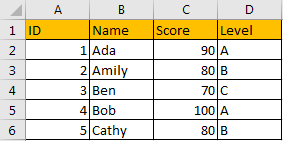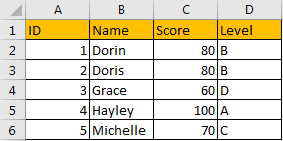Suppose we want to create filter on Level column to filter student whose level is ‘A’, how can we create filter on two worksheets simultaneously?

## Method: Create Filter on Multiple Worksheets Via VBA Code

Step 1: On current visible worksheet, right click on sheet name tab to load Sheet management menu. Select View Code, Microsoft Visual Basic for Applications window pops up.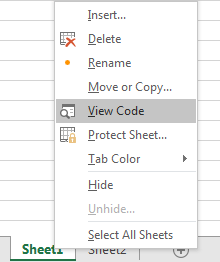Or you can enter Microsoft Visual Basic for Applications window via Developer->Visual Basic. You can also press Alt + F11 keys simultaneously to open it.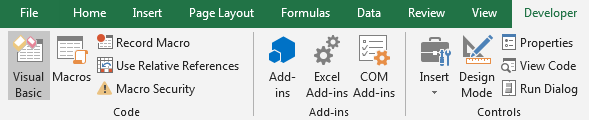Step 2: In Microsoft Visual Basic for Applications window, click Insert->Module, enter below code in Module1:

```Sub autofilter1()

Dim ws As Worksheet

On Error Resume Next

For Each ws In Worksheets

ws.Range("\$D\$1").autofilter 4, "=A"

Next

End Sub```

\$D\$1 indicates the position (or column) we want to create filter for; autofilter 4 indicates the number of column, if we want to filter on column A, here we type autofilter 1; ‘=A’ is the criteria.

Step 3: Save code, see screenshot below.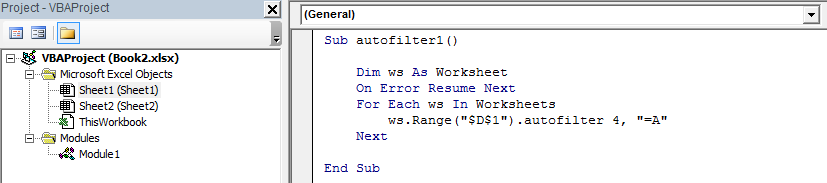Step 4: Don’t quit current page, press F5 to trigger Macro directly.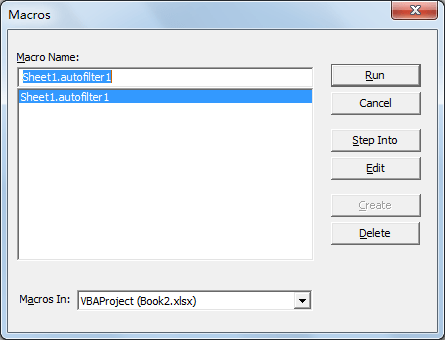If you already quit current page, you can click Developer->Macros to run Macro.

Step 5: Select Sheet1.autofilter1, click Run.

Step 6: Quit Microsoft Visual Basic for Applications window. Verify that filters are created on both worksheet1 and worksheet2. Level A is filtered properly on two worksheets.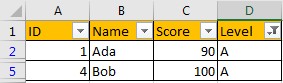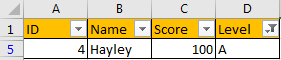Comment:

1. If we want to filter where score>=80, we can enter below code:
```Sub autofilter2()

Dim ws As Worksheet

On Error Resume Next

For Each ws In Worksheets

ws.Range("\$C\$1").autofilter 3, ">=80"

Next

End Sub```

Run F5 and verify filter is applied on two worksheets properly.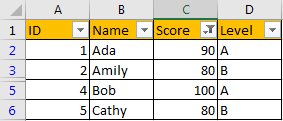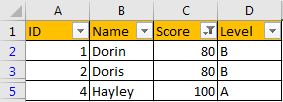Related Posts

Find and Replace Multiple Values

This post will guide you how to find and replace multiple values at once with VBA macro or using formula  in Excel. How do I make multiple find and replace in Excel. Suppose that you have a few cells containing ...

VBA Macro For VLOOKUP From Another Sheet

In the previous post, you should know that how to fix or remove the #N/A error when using VLOOKUP formula to lookup value from another sheet. And this post will show you how to use VBA code to vlookup data ...

How To Insert Comments in Protected Worksheet in Excel

This post will show you how to allow comments in a protected worksheet in Excel. You can easily to insert comments into cells in a normal worksheet in Excel, but if want to insert a comment in a worksheet that ...

How To Convert Text to Upper Cases(Using VBA) in Excel

This post will show you how to switch from lower case to upper case in Excel. and I am going to show you two different ways of converting text to upper cases using formula or VBA macro in Excel 2013,Excel ...

How To Hide Every Other Row in Excel (Using VBA)

This post will show you how to hide alternate rows or columns in Excel or how to hide every third, fourth, fifth row or column in Excel. If you want to hide every other row in your current worksheet, how ...

How to Disable the Save As Prompt in Excel

This post will show you how to use a VBA Macro to save an Excel file and overwrite any existing file without a prompt so that you are going to get the little window that says file already exists do ...

How to Count Cells that Contain even or odd numbers in Excel

This post will guide you how to count the number of cells that contain odd or even numbers within a range of cells using a formula in Excel 2013/2016.How do I count cells that contain odd numbers through the use ...

How to Count Cells that Contain negative Numbers in Excel

This post will guide you how to count the number of cells that contain negative numbers within a range of cells using a formula in Excel 2013/2016.You can count the number of negative numbers in your data using easy functions ...

How to Count Cells Are Not Blank or Empty in Excel

This post will guide you how to count cells that are not blank or empty in a given range cells using a formula in Excel 2013/2016.How do I count the number of cells that are not blank in a particular ...

How to Count Cells Less Than a Specific Value in Excel

This post will guide you how to count the number of cells less than a particular numeric value in a given range cells using a formula in Excel 2013/2016. How do I count cells that are less than a specific ...

Sidebar